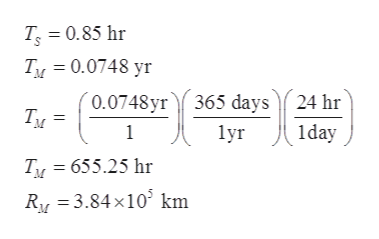Question
101 views

Problem 5: Suppose you are told that a satellite orbiting the Earth has a orbital period of 0.85 hours.

a)Using the orbital characteristics of the Moon (RM = 3.84 × 105km and TM = 0.0748 y), use Kepler's laws to calculate the orbital radius for the satellite, in kilometers.

MultipleChoice   :

1) This radius is unreasonable because it is smaller than the orbital radius of the Moon.
2) There is nothing unreasonable about the result.
3) This radius is unreasonable because it is greater than the radius of the Earth.
4) This radius is unreasonable because it is greater than the orbital radius of the Moon.
5) This radius is unreasonable because it is smaller than the radius of Earth.

check_circle

Step 1

Given,help_outlineImage TranscriptioncloseТ, 3 0.85 hr Ty 0.0748 yr 0.0748yr365 days24 hr Tи 1 lyr 1day Tu 655.25 hr RM 3.84 x10 km fullscreen
Step 2

Part(a)

Applying Kepler’s third law, which states that the square of the period of orbit is proportional to the cube of the semi major axis of its orbit. i.e.:

Step 3

Now, if TS is the time period of the satellite and TM is the time period o...

### Want to see the full answer?

See Solution

#### Want to see this answer and more?

Solutions are written by subject experts who are available 24/7. Questions are typically answered within 1 hour.*

See Solution
*Response times may vary by subject and question.
Tagged in

### Gravitation